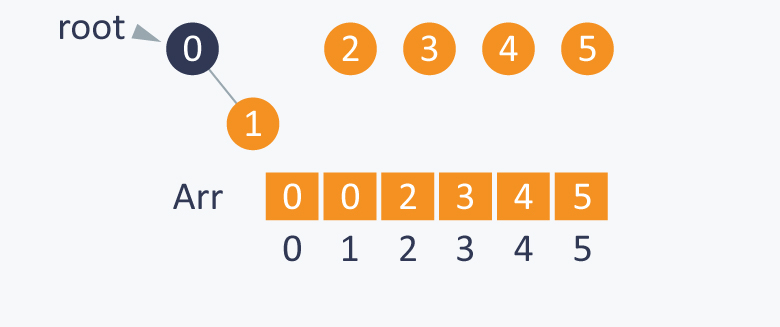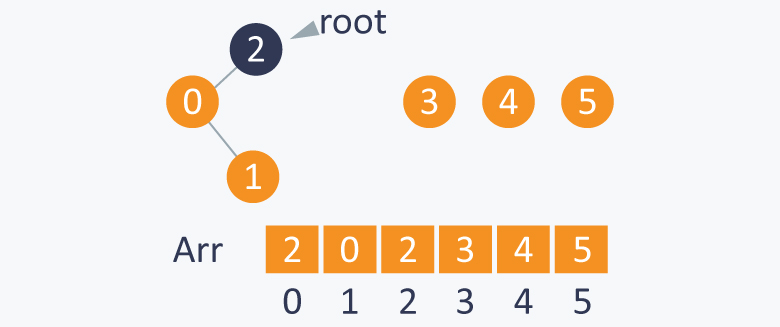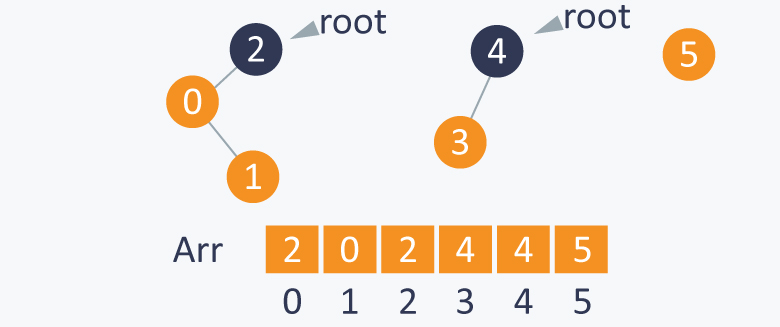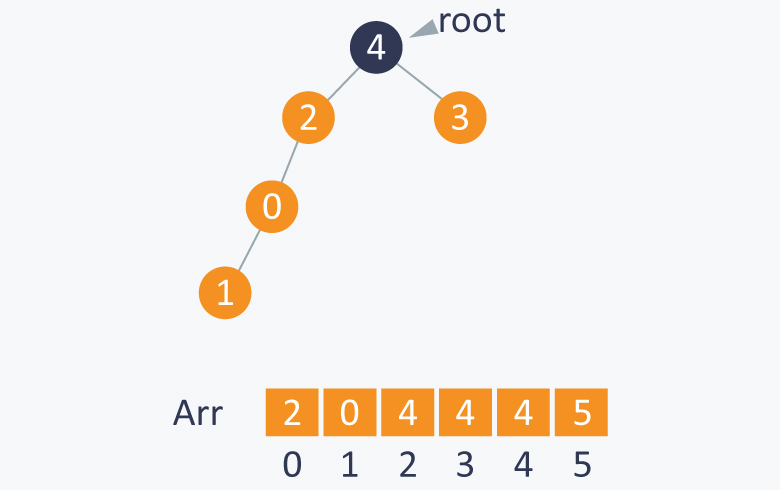# Disjoint Data Structures

Let's try another approach.

Idea

Arr[ A ] is a parent of A.

Consider the root element of each subset, which is only a special element in that subset having itself as the parent. Assume that R is the root element, then arr[ R ] = R.

For more clarity, consider the subset S = {0, 1, 2, 3, 4, 5}

Initially each element is the root of itself in all the subsets because arr[ i ] = i, where i is the element in the set. Therefore root(i) = i.Performing union(1, 0) will connect 1 to 0 and will set root (0) as the parent of root (1). As root(1) = 1 and root(0) = 0, the value of arr[ 1 ] will change from 1 to 0. Therefore, 0 will be the root of the subset that contains the elements {0, 1}.Performing union (0, 2), will indirectly connect 0 to 2 by setting root(2) as the parent of root(0). As root(0) is 0 and root(2) is 2, it will change value of arr[ 0 ] from 0 to 2. Therefore, 2 will be the root of the subset that contains the elements {2, 0, 1}.Performing union (3, 4) will indirectly connect 3 to 4 by setting root(4) as the parent of root(3). As root(3) is 3 and root(4) is 4, it will change the value of arr[ 3 ] from 3 to 4. Therefore, 4 will be the root of the subset that contains the elements {3, 4}.Performing union (1, 4) will indirectly connect 1 to 4 by setting root(4) as the parent of root(1). As root(4) is 4 and root(1) is 2, it will change value of arr[ 2 ] from 2 to 4. Therefore, 4 will be the root of the set containing elements {0, 1, 2, 3, 4}.After each step, you will see the change in the array arr also.

After performing the required union(A, B) operations, you can perform the find(A, B) operation easily to check whether A and B are connected. This can be done by calculating the roots of both A and B. If the roots of A and B are the same, then it means that both A and B are in the same subset and are connected.

Calculating the root of an element

Arr[ i ] is the parent of i (where i is the element of the set). The root of i is Arr[ Arr[ Arr[ ...Arr[ i ]...... ] ] ] until arr[ i ] is not equal to i. You can run a loop until you get an element that is a parent of itself.

Note This can only be done when there is no cycle in the elements of the subset, else the loop will run infinitely.

1. Find(1, 4): 1 and 4 have the same root i.e. 4. Therefore, it means that they are connected and this operation will give the result True.

2. Find(3, 5): 3 and 5 do not have same root because root(3) is 4 and root(5) is 5. This means that they are not connected and this operation will give the result False.

Implementation

Initially each element is a parent of itself, which can be done by using the initialize function as discussed above.

``  //finding root of an element    int root(int Arr[ ],int i)    {        while(Arr[ i ] != i)           //chase parent of current element until it reaches root        {         i = Arr[ i ];        }        return i;    }    /*modified union function where we connect the elements by changing the root of one of the elements*/    int union(int Arr[ ] ,int A ,int B)    {        int root_A = root(Arr, A);               int root_B = root(Arr, B);          Arr[ root_A ] = root_B ;       //setting parent of root(A) as root(B)    }    bool find(int A,int B)    {        if( root(A)==root(B) )       //if A and B have the same root, it means that they are connected.        return true;        else        return false;    }``
In the worst case, this idea will also take linear time in connecting 2 elements and determining (finding) whether two elements are connected. Another disadvantage is that while connecting two elements, which subset has more elements is not checked. This may sometimes create a big problem because you will have to perform approximately linear time operations.
Implementation of above approach:
#include<iostream>
using namespace std;
void initialize( int Arr[ ], int N)
{
for(int i = 0;i<N;i++)
Arr[ i ] = i ;
}
//returns true if A and B are connected, else returns false
//finding root of an element
int root(int Arr[ ],int i)
{
while(Arr[ i ] != i) //chase parent of current element until it reaches root
{
i = Arr[ i ];
}
return i;
}

/*modified union function where we connect the elements by changing the root of one of the elements*/

void unionr(int Arr[ ] ,int A ,int B)
{
int root_A = root(Arr, A);
int root_B = root(Arr, B);
Arr[ root_A ] = root_B ; //setting parent of root(A) as root(B)
}
bool find(int * a,int A,int B)
{
if( root(a,A)==root(a,B) ) //if A and B have the same root, it means that they are connected.
return true;
else
return false;
}
int main(){
int a,n=10;
initialize(a,n);
cout<<"Printing array entries:\n";
for(int i=0;i<n;i++){
cout<<a[i]<<" ";
}
cout<<endl;
cout<<"Checking whether 2 and 3 belongs to same array:\n";
if(!find(a,2,3)){
cout<<"No";
}
else{
cout<<"Yes";
}
cout<<endl;
cout<<"Making 2 and 3 to be in same group\n";
unionr(a,2,3);
cout<<"Checking whether 2 and 3 belongs to same array:\n";
if(!find(a,2,3)){
cout<<"No";
}
else{
cout<<"Yes";
}
cout<<endl;

}
Output:
`Printing array entries:0 1 2 3 4 5 6 7 8 9Checking whether 2 and 3 belongs to same array:NoMaking 2 and 3 to be in same groupChecking whether 2 and 3 belongs to same array:Yes`#### More Articles of M Mounika:

Name Views Likes
C++ Segmented Sieve (Print Primes In a Range) 162 0
C++ Sieve Of Erastosthenes 136 0
C++ Gold Mine Problem 295 0
C++ Merge K Sorted Arrays 117 0
C++ K Centers Problem 240 0
C++ Find Nth Catalan Number 311 0
C++ Inplace Rotate square matrix by 90 degrees 286 0
C++ Find Non Repeating Elements in Array 87 0
C++ Merge Two Binary Trees 121 0
C++ Sum of Numbers From Root To Leaf Paths 89 0
C++ Meta Strings 92 0
C++ Flood Fill Algorithm 402 0
C++ smallest substring with maximum distinct characters 200 0
C++ Smallest window with all characters in string 94 0
C++ Minimum Removal of Characters from string to make its permutation as palindrome 87 0
C++ Minimum characters added at front of string in palindrome conversion 70 0
C++ Number of Bracket Reversals needed to make expression Balanced 72 0
C++ String to Palindrome with Append Function 83 0
C++ WildCard pattern matching 76 0
C++ Anagram substring Search 72 0
C++ Manachars Algorithm 74 0
C++ Search String in Grid 83 0
C++ String Matching(Z Algorithm) 67 0
C++ String Matching(Naive Algorithm) 114 0
C++ String Matching(KMP Algorithm) 141 0
C++ Remove Duplicates From String 111 0
C++ Basics of String Manipulation 85 1
C++ Disjoint Data Structure Cycle Detection 87 0
C++ Problem On Disjoint Data Structures 95 0
C++ Disjoint Data Structures Part3 79 0
Disjoint Data Structures Part2 91 0
Disjoint Data Structures 93 1
C++ Segment Trees 321 2
C++ Trie Cost of Data 291 1
C++ Trie Datastructure 279 1
C++ Greedy Approach Minimum number of coins 526 0
C++ Greedy Approach Maximum height Pyramid 329 1
C++ Greedy Approach String lexicographically largest subsequence 247 0
C++ Greedy Approach Lexicographically largest subsequence 364 0
C++ Greedy Approach Prims MST 398 1
C++ Greedy Approach Krushkals MST 458 1
C++ Greedy Approach N-array maximum sum 334 1
C++ Greedy Approach Policemen Catch Thieves 563 1
C++ Greedy Approach Maximum product Subset 546 1
C++ Greedy Approach Minimum Product Subset 349 1
C++ Greedy Approach Fractional Knapsack 737 1
C++ Greedy Approach-Activity Selection Problem 745 1
C++ Greedy Approach-Egyptian Fractions 640 0
C++ Greedy Approach-Huffman Codes 1031 1
C++ Introduction to Greedy Approach 955 2